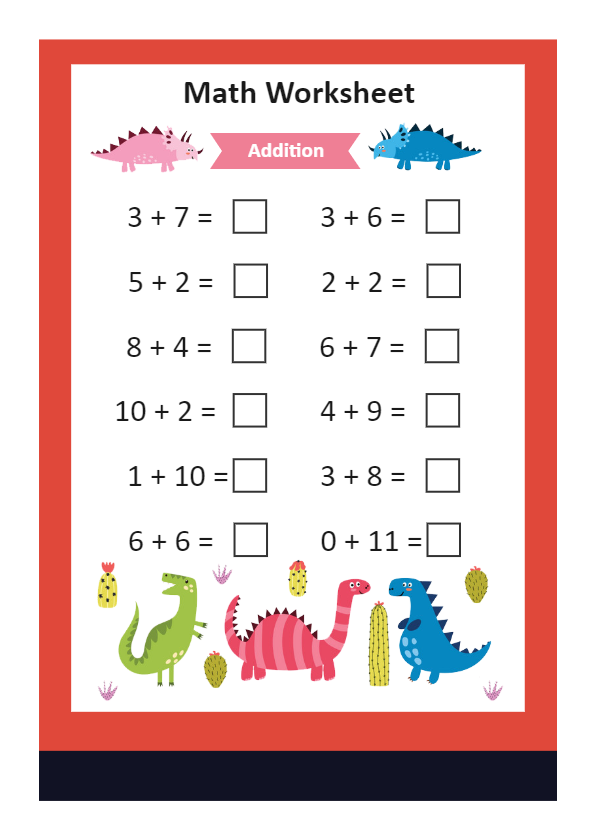Template Community / Math Worksheet Addition ExampleKiraaaa
Published on 2022-02-09
Edit OnlineFollowing math worksheet shows the additional process between two numbers. Students will be given this additional math worksheet, and they will have to enter the right digits in the empty columns. Once they submit it, teachers will understand how well the students solve the additional problems. In addition to this, teachers can share this additional math worksheet with the parents using EdrawMax's in-built sharing feature. As you can see here, math is explained in easy language, so the preschool and kindergarten students will not have difficulty understanding the basic concept of addition. You can use EdrawMax to create math worksheet templates for addition and subtraction problems.
Tag
Worksheet
Report
0
21PostRecommended Templates